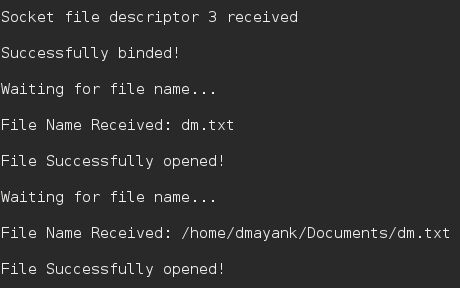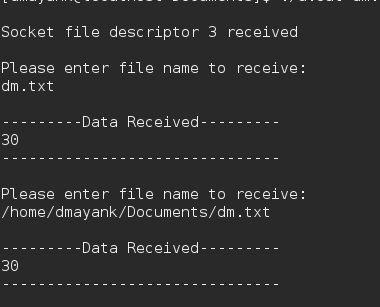Related Articles

# C program for file Transfer using UDP

• Difficulty Level : Medium
• Last Updated : 30 Jul, 2019

Data can be transferred between two computers using Socket programming in C.

Similarly, files can easily be sent using UDP protocol and a simple client/server.

Security: Handled by encryption.

Protocol : UDP
Encryption: XOR encryption

Algorithm :

```1. The server starts and waits for filename.
2. The client sends a filename.
If file is present,
and continues to send a buffer filled with
file contents encrypted until file-end is reached.
4. End is marked by EOF.
5. File is received as buffers until EOF is
```

The Server :

 `// server code for UDP socket programming``#include ``#include ``#include ``#include ``#include ``#include ``#include ``#include `` ` `#define IP_PROTOCOL 0``#define PORT_NO 15050``#define NET_BUF_SIZE 32``#define cipherKey 'S'``#define sendrecvflag 0``#define nofile "File Not Found!"`` ` `// function to clear buffer``void` `clearBuf(``char``* b)``{``    ``int` `i;``    ``for` `(i = 0; i < NET_BUF_SIZE; i++)``        ``b[i] = ``'\0'``;``}`` ` `// function to encrypt``char` `Cipher(``char` `ch)``{``    ``return` `ch ^ cipherKey;``}`` ` `// function sending file``int` `sendFile(``FILE``* fp, ``char``* buf, ``int` `s)``{``    ``int` `i, len;``    ``if` `(fp == NULL) {``        ``strcpy``(buf, nofile);``        ``len = ``strlen``(nofile);``        ``buf[len] = EOF;``        ``for` `(i = 0; i <= len; i++)``            ``buf[i] = Cipher(buf[i]);``        ``return` `1;``    ``}`` ` `    ``char` `ch, ch2;``    ``for` `(i = 0; i < s; i++) {``        ``ch = ``fgetc``(fp);``        ``ch2 = Cipher(ch);``        ``buf[i] = ch2;``        ``if` `(ch == EOF)``            ``return` `1;``    ``}``    ``return` `0;``}`` ` `// driver code``int` `main()``{``    ``int` `sockfd, nBytes;``    ``struct` `sockaddr_in addr_con;``    ``int` `addrlen = ``sizeof``(addr_con);``    ``addr_con.sin_family = AF_INET;``    ``addr_con.sin_port = htons(PORT_NO);``    ``addr_con.sin_addr.s_addr = INADDR_ANY;``    ``char` `net_buf[NET_BUF_SIZE];``    ``FILE``* fp;`` ` `    ``// socket()``    ``sockfd = socket(AF_INET, SOCK_DGRAM, IP_PROTOCOL);`` ` `    ``if` `(sockfd < 0)``        ``printf``(``"\nfile descriptor not received!!\n"``);``    ``else``        ``printf``(``"\nfile descriptor %d received\n"``, sockfd);`` ` `    ``// bind()``    ``if` `(bind(sockfd, (``struct` `sockaddr*)&addr_con, ``sizeof``(addr_con)) == 0)``        ``printf``(``"\nSuccessfully binded!\n"``);``    ``else``        ``printf``(``"\nBinding Failed!\n"``);`` ` `    ``while` `(1) {``        ``printf``(``"\nWaiting for file name...\n"``);`` ` `        ``// receive file name``        ``clearBuf(net_buf);`` ` `        ``nBytes = recvfrom(sockfd, net_buf,``                          ``NET_BUF_SIZE, sendrecvflag,``                          ``(``struct` `sockaddr*)&addr_con, &addrlen);`` ` `        ``fp = ``fopen``(net_buf, ``"r"``);``        ``printf``(``"\nFile Name Received: %s\n"``, net_buf);``        ``if` `(fp == NULL)``            ``printf``(``"\nFile open failed!\n"``);``        ``else``            ``printf``(``"\nFile Successfully opened!\n"``);`` ` `        ``while` `(1) {`` ` `            ``// process``            ``if` `(sendFile(fp, net_buf, NET_BUF_SIZE)) {``                ``sendto(sockfd, net_buf, NET_BUF_SIZE,``                       ``sendrecvflag, ``                    ``(``struct` `sockaddr*)&addr_con, addrlen);``                ``break``;``            ``}`` ` `            ``// send``            ``sendto(sockfd, net_buf, NET_BUF_SIZE,``                   ``sendrecvflag,``                ``(``struct` `sockaddr*)&addr_con, addrlen);``            ``clearBuf(net_buf);``        ``}``        ``if` `(fp != NULL)``            ``fclose``(fp);``    ``}``    ``return` `0;``}`

The Client:

 `// client code for UDP socket programming``#include ``#include ``#include ``#include ``#include ``#include ``#include ``#include `` ` `#define IP_PROTOCOL 0``#define IP_ADDRESS "127.0.0.1" // localhost``#define PORT_NO 15050``#define NET_BUF_SIZE 32``#define cipherKey 'S'``#define sendrecvflag 0`` ` `// function to clear buffer``void` `clearBuf(``char``* b)``{``    ``int` `i;``    ``for` `(i = 0; i < NET_BUF_SIZE; i++)``        ``b[i] = ``'\0'``;``}`` ` `// function for decryption``char` `Cipher(``char` `ch)``{``    ``return` `ch ^ cipherKey;``}`` ` `// function to receive file``int` `recvFile(``char``* buf, ``int` `s)``{``    ``int` `i;``    ``char` `ch;``    ``for` `(i = 0; i < s; i++) {``        ``ch = buf[i];``        ``ch = Cipher(ch);``        ``if` `(ch == EOF)``            ``return` `1;``        ``else``            ``printf``(``"%c"``, ch);``    ``}``    ``return` `0;``}`` ` `// driver code``int` `main()``{``    ``int` `sockfd, nBytes;``    ``struct` `sockaddr_in addr_con;``    ``int` `addrlen = ``sizeof``(addr_con);``    ``addr_con.sin_family = AF_INET;``    ``addr_con.sin_port = htons(PORT_NO);``    ``addr_con.sin_addr.s_addr = inet_addr(IP_ADDRESS);``    ``char` `net_buf[NET_BUF_SIZE];``    ``FILE``* fp;`` ` `    ``// socket()``    ``sockfd = socket(AF_INET, SOCK_DGRAM,``                    ``IP_PROTOCOL);`` ` `    ``if` `(sockfd < 0)``        ``printf``(``"\nfile descriptor not received!!\n"``);``    ``else``        ``printf``(``"\nfile descriptor %d received\n"``, sockfd);`` ` `    ``while` `(1) {``        ``printf``(``"\nPlease enter file name to receive:\n"``);``        ``scanf``(``"%s"``, net_buf);``        ``sendto(sockfd, net_buf, NET_BUF_SIZE,``               ``sendrecvflag, (``struct` `sockaddr*)&addr_con,``               ``addrlen);`` ` `        ``printf``(``"\n---------Data Received---------\n"``);`` ` `        ``while` `(1) {``            ``// receive``            ``clearBuf(net_buf);``            ``nBytes = recvfrom(sockfd, net_buf, NET_BUF_SIZE,``                              ``sendrecvflag, (``struct` `sockaddr*)&addr_con,``                              ``&addrlen);`` ` `            ``// process``            ``if` `(recvFile(net_buf, NET_BUF_SIZE)) {``                ``break``;``            ``}``        ``}``        ``printf``(``"\n-------------------------------\n"``);``    ``}``    ``return` `0;``}`

Output :
Server :Client :Attention reader! Don’t stop learning now. Get hold of all the important CS Theory concepts for SDE interviews with the CS Theory Course at a student-friendly price and become industry ready.

My Personal Notes arrow_drop_up